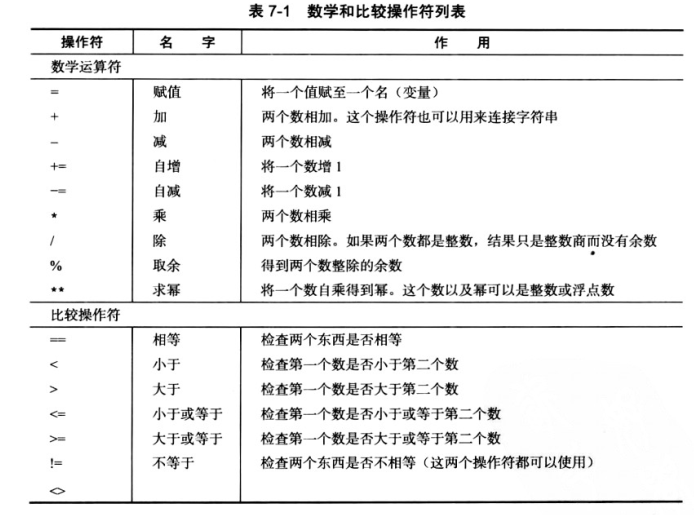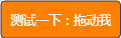# Python数学运算### 1.数字的加减乘除

``````print(5+2)
print(5-2)

print(5*2)
print(3**3)   # 幂 27 **号代表3的3次幂，也就是3*3*3

print(5/3)
print (format(5/3,".1f")) #通过`".1f"`控制小数的位数显示
print(7%2)    #取余数：1，%可以拿来取余数
``````

### 2.字符的加乘

``````print("a"+"b")
print("a"*2) #字符无法做减法与除法``````

### 3.科学计数法

``````a=3.8
b=10**16
print (a*b)``````